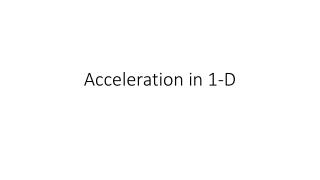# Acceleration in 1-D - PowerPoint PPT PresentationDownload PresentationAcceleration in 1-D

Acceleration in 1-DDownload Presentation## Acceleration in 1-D

- - - - - - - - - - - - - - - - - - - - - - - - - - - E N D - - - - - - - - - - - - - - - - - - - - - - - - - - -
##### Presentation Transcript

1. Acceleration in 1-D

2. What causes acceleration? What is acceleration? Change in velocity This includes a change in magnitude or direction What causes acceleration? A Force

3. → → • aav = Δv Δt → where aav = average acceleration → Δv = change in velocity Δt = time

4. → → j = Δa Δt → where j = jerk (constant) → Δa = change in acceleration Note: jerk is not a desired type of motion, so usually it is not analyzed.

5. Equations • For any accelerated motion, we use the constant acceleration equations

6. Kinetic Equations: → → → • v2 = v1 + a Δt → → → 2. Δd = (v2 – v1) Δt 2

7. Kinetic Equations: → → → 3. Δd = v1 + 0.5 a Δt2 → → → 4. Δd = v2 – 0.5 a Δt2 → → → → 5. v22 = v12 + 2 a Δd

8. Example #1: An SUV that goes 60 m [fwd] as it stops. The magnitude of the stopping acceleration is 3.0 m/s2. • What is the initial velocity? • What is the stopping time?

9. Acceleration in 2-D • Since these are vectors, we must use vector subtraction • In other words, when we add vectors we place them head to tail • In order to subtract vectors, we reverse the direction of the negative vector and then add the two vectors

10. Example #2: (2D-acceleration) A car goes around a turn with a constant acceleration. The car was going 15m/s [N] as it entered the turn and came out at 15m/s [W]. If the turn lasted 10 seconds, what is the acceleration around the turn?

11. Example # 3 Arnav travels at 34.5 m/s [E 20o N]. Over a period of 5.00s, he changes his velocity to 34.5 [W 32oN]. What is Arnav’s acceleration?